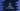# Two different C++ program to find the largest of n numbers## C++ program to find the largest of n numbers:

In this post, we will learn how to find the largest of n user input numbers in C++. The program will ask the user to enter the value of n, i.e. total numbers to check. It will then take the numbers one by one from the user. Finally, it will print the largest of all the user input numbers.

This program can be solved in two ways. We can read the numbers and store them in an array and iterate this array elements one by one to find out the largest.

I will show you both of these ways to solve this problem. Let’s have a look:

### C++ program to find the largest of n numbers using an array:

Let’s see how to find the largest of n numbers using an array. We will keep these values in an array and find out the largest value by iterating through the elements.

Below is the complete C++ program:

``````#include <iostream>
using namespace std;

int main()
{
int size;

cout << "Enter total numbers you want to add :" << endl;
cin >> size;

int *arr = new int(size);

for (int i = 0; i < size; i++)
{
cout << "Enter value to add : ";
cin >> *(arr + i);
}

int largestValue = *arr;

for (int i = 1; i < size; i++)
{
if (*(arr + i) > largestValue)
{
largestValue = *(arr + i);
}
}

cout << "Largest value : " << largestValue << endl;
return 0;
}``````

### Explanation:

Here,

• It is reading the total numbers in the variables size
• Using a for loop, it is reading all numbers and adding it to the array arr.
• largestValue is a variable defined by initializing with the first number of arr.
• The second for loop iterates through the elements of the array one by one starting from the second element. For each element, it compares it with largestValue. If the current element is larger than largestValue, it updates largestValue’s value as this element. At the end of the program, largestValue holds the largest of all numbers in the array.

### Sample output:

If you execute the above program, it will print output as like below:

``````Enter total numbers you want to add :
5
Enter value to add : 1
Enter value to add : 2
Enter value to add : 3
Enter value to add : 4
Enter value to add : 5
Largest value : 5

Enter total numbers you want to add :
4
Enter value to add : 10
Enter value to add : 200
Enter value to add : 20
Enter value to add : 1000
Largest value : 1000``````

### Method 2: Find out the largest number without using an array:

Using an array requires extra space. Also, we are using two for loops in the above example. So, we are iterating through the array two times, which is not efficient.

Instead, we can also find out the largest value without using an array. For that, we will do the comparison when user is entering the values. i.e. for each value or number user enters, compare it with the current largest value. In short:

• For the first number entered, assign it to a variable to hold the largest value.
• For second and other numbers, compare it with the current largest value and update the largest value accordingly.

Below is the complete C++ program:

``````#include <iostream>
using namespace std;

int main()
{
int size, largestValue, currentValue;

cout << "Enter total numbers you want to add :" << endl;
cin >> size;

for (int i = 0; i < size; i++)
{
cout << "Enter value to add : ";
cin >> currentValue;

if (i == 0 || currentValue > largestValue)
{
largestValue = currentValue;
}
}

cout << "Largest value : " << largestValue << endl;
return 0;
}``````

Here,

• currentValue variable is used to hold the current value user entered.
• largestValue holds the largest value of all entered numbers.
• If the entered number is the first number, we are assigning it to largestValue. Else, we are comparing all other numbers to largestValue and if it is more than largestValue, assign largestValue’s value as this number.

If you run this program, it will give similar output as the above program:

``````Enter total numbers you want to add :
4
Enter value to add : 20
Enter value to add : 200
Enter value to add : 10
Enter value to add : 2222
Largest value : 2222``````

Both of these methods are useful in any applications. If you are reading the user inputs than second method is more preferred. You can go with any of these examples based on your requirement.